Next: Results Up: Artificial data Previous: Artificial data

## Methods

For all but the Gaussian activation artificial dataset (figure 6) we use artificial data generated by sampling from the mixture component distributions described in the last section, with the data deactivation parameters set toand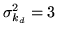, and the activation parameters set to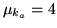and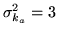. For all artificial datasets (including the Gaussian activation artificial dataset), the non-activation parameters were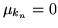and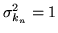.

Unlike the other artificial datasets, the Gaussian activation artificial dataset (figure 6) is not generated fully from the model. This is because the activation and deactivation were not sampled from the mixture component distributions, but were instead modelled as 2-D spatial Gaussians added to non-activation. This dataset is included to see how the model deals with data not generated from the model. The 2-D spatial Gaussians used have a maximum peak of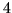for the activation and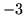for the deactivation, and a diagonal covariance matrix equal to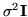, where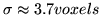for both activation and deactivation. These 2-D spatial Gaussians were added to samples from the non-activation distribution

In all cases we generate a 2-dimensional dataset with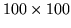pixels. The different spatial patterns of the five different artificial datasets are shown in the top right of figures 3--7.

The models we infer upon are the three models described in section 4. Model 2 is interesting as it is similar to those proposed in Zhang et al. (2001); Salli et al. (1999), in that the spatial smoothness is non-adaptive. Instead the MRF spatial smoothness parameter is arbitrarily fixed at a value of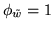. This will act as a comparison for Model 3 where the spatial regularisation is adaptively determined.Next: Results Up: Artificial data Previous: Artificial data